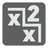Elementary+
English RU

In mathematical numeral systems, the radix or base is the number of unique digits (including zero) that a positional numeral system uses to represent numbers. For example: in the decimal system, the radix is ten. This is because it has ten digits from 0 through 9. In a system with a radix of 13, there would be 13 digits. For example: a string of digits such as 398 denotes the decimal number 3 * 13^2 + 9 * 13^1 + 8 * 13^0 . For the systems with a radix more than 10, we will use capital Latin symbols from A to Z, where A = 10, B = 11 ...

You are given some number n written as a string with the radix equal to k (1 < k < 37) . We know that our number is divisible by (k - 1) without a remainder. You should find the minimal possible k , if it exists, or return 0.

For example: n = "18". As we can see k should be greater than 8.
If k == 9, then n = 17 in the decimal system and 17 % 8 == 1. The wrong radix.
If k == 10, then n = 18 in the decimal system and 18 % 9 == 0. We found the answer.

Input: A number as a string.

Output: The radix k as an integer.

```checkio(u"18") == 10
checkio(u"1010101011") == 2
checkio(u"222") == 3
checkio(u"A23B") == 14
checkio(u"IDDQD") == 0
```

How it is used: Let's familiarize with numeral systems more careful and examine the radix. Many devices are built to accept numbers in decimal representation and display results in decimal. Often such devices convert from decimal to some internal radix on input, do all internal operations in that radix, and then convert the results from the internal radix to decimal on output. Such devices could in principle use any radix internally. The people who design such computing devices sometimes wonder what would be the "best" radix to use internally -- radix economy. The octal, hexadecimal and base-64 systems are often used in computing because of their ease as shorthand for binary.

Precondition: A number conforms regexp "[A-Z0-9]{1, 10}".
0 < number45
Settings
Code:
Other:
Invalid hot key. Each hot key should be unique and valid
Hot keys:
•  to Run Code: to Check Solution: to Stop:
CheckiO Extensions

CheckiO Extensions allow you to use local files to solve missions. More info in a blog post.

In order to install CheckiO client you'll need installed Python (version at least 3.8)

Install CheckiO Client first:

`pip3 install checkio_client`

`checkio --domain=py config --key=`

Sync solutions into your local folder

`checkio sync`

(in beta testing) Launch local server so your browser can use it and sync solution between local file end extension on the fly. (doesn't work for safari)

`checkio serv -d`

Alternatevly, you can install Chrome extension or FF addon

`checkio install-plugin`
`checkio install-plugin --ff`
`checkio install-plugin --chromium`

Read more here about other functionality that the checkio client provides. Feel free to submit an issue in case of any difficulties.